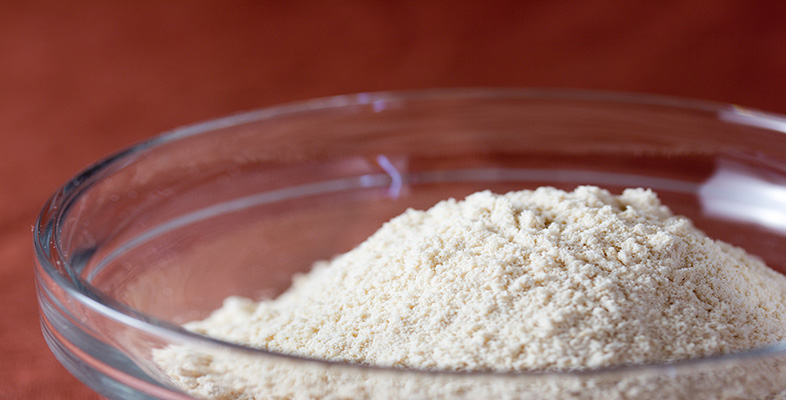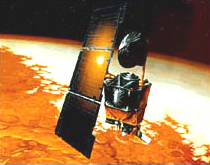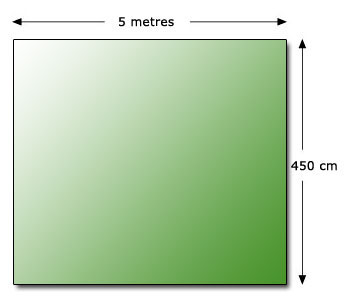Rounding and estimation

This free course is available to start right now. Review the full course description and key learning outcomes and create an account and enrol if you want a free statement of participation.

Free course

# 3.3 Have I given due consideration to units of measurement?

Many mathematical problems include units of measurement. The measurement may be of length, weight, time, temperature or currency. The UK uses both metric and imperial units.

The table below gives the units of length that are in everyday use in the UK, but you may know some others.

 Metric Imperial millimetre inches centimetre feet metre yards kilometre miles

There is a great variety of measurement units in use. Their incorrect use leads to errors which can sometimes be costly.

On 23 September 1999, NASA lost a \$125 million Mars orbiter. This was because an engineering team used imperial units of measurement while NASA's team used the metric system for a key spacecraft operation.## Example 10Suppose that you want to buy a carpet, priced at £16.00 per square metre, for the room shown above and you calculate the area as follows.

Area = 5 × 450 = 2250

Cost of carpet = 16 × 2250 = £3600

An expensive carpet! What is wrong?

There is a mixture of units: metres and centimetres.

If a problem has a mixture of measurement units, it is usually a good idea to convert all measures to the same units. Here the carpet is sold in units of ‘square metres’, therefore ensure all the measurements are in metres.

Hence area required = 5 × 4.50.

Area = 22.5 square metres.

Cost of carpet = £16 × 22.5 = £360.

MU120_4M2